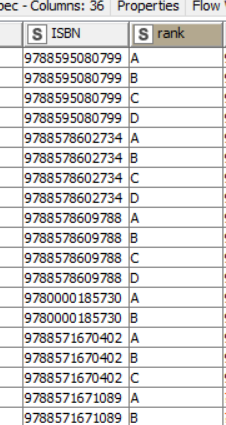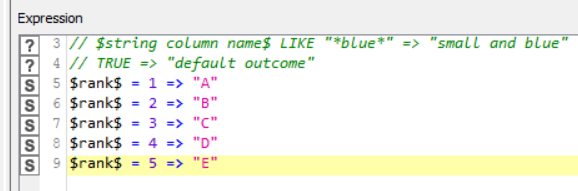# Math Operations on Letters

Is there possible to do math operations with letters? Is There a node or a couple of nodes that could perform such operations?

For example, ‘A’ + 1 = ‘B’ or ‘A’ + 3 = ‘D’ or ‘Z’ + 1 = ‘AB’, just like excel.
Thanks.

not that I know of. And what would be a use case behind it?

Br,
Ivan

Hi,

You can assign numbers to letters (A=1, B=2,…) a kind of dictionary, do the calculation and then transform back to numbers A+2 = A+B = 3 = C …
I do not understand why ‘Z’ + 1 = ‘AB’, why is not ‘A’ … what is AB+1 = ?? (BB or AC)

Hi! Sorry for delay.

This is the cenario: I have a column called ISBN with repeated values. So I used a Rank Node grouping ISBN and then I used a Rule Engine Node to associate value A to 1, B to 2 and so on… in my case, the number of repeated ISBN are limited, so I could se the max number of repetitions and assingn the maximum value (in my case, E). So, my idea is to upgrade the solution to considerer a higher number of ISBN repeated values. So, the idea is to have some mechanism to take the max value and assign a alphabet letter correspondingly. A possible solution that I have imagined is to take as a start a table containing one record only (value: A) and then add the max number of repeated values to have de final letter.

Thanks for the attention.

Hi @rafaelesanto83,
what do you mean with repeated ISBN? Do you mean the same ISBN occurs n times and you want to count the maximum number each ISBN occurs? If that is the case you can solve this easy with the group by node. If this is not the case please profide an example with case data to get a better understanding.

BR

Hi @morpheus,

In this case I want to assign a new value to repeated ISBN. I´m sending some images of Rule Engine Node and the result. I´m wondering if there is a generic way to assingn the letters.Hi @rafaelesanto83,
maybe java Character.toString(counter+64) does the job. But consider there are still limitations using characters for ranking.

BR

This topic was automatically closed 182 days after the last reply. New replies are no longer allowed.